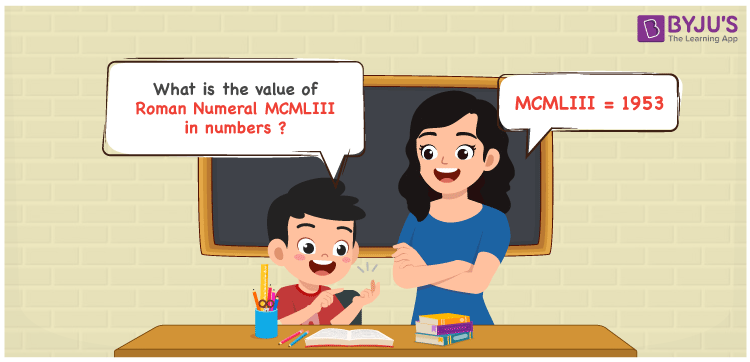Checkout JEE MAINS 2022 Question Paper Analysis : Checkout JEE MAINS 2022 Question Paper Analysis :

# MCMLIII Roman Numerals

MCMLIII Roman Numerals is 1953. Roman Numerals are the letters used by the ancient Romans to express numbers. Roman Numerals Conversion in PDF format is the best tool to learn the conversion of Roman Numerals into numbers in a better way. This also helps students to grasp the in-depth knowledge based on Roman letters. Let us have a look at how to represent the numerical value of MCMLIII Roman Numerals in this article.

 Number Roman Numeral 1953 MCMLIII

## How to Write MCMLIII Roman Numerals?To represent the Roman Numeral MCMLIII into numbers, we use any of the two methods given below

First Method:

• Break the Roman Numerals into single letters
• MCMLIII = M + (M – C) + L + I + I + I
• Write the numerical value of each letter and add/subtract them
• MCMLIII = 1000 + (1000 – 100) + 50 + 1 + 1 + 1 = 1953

Second Method:

In this method, we consider the groups of Roman Numerals for addition or subtraction

• MCMLIII = M + CM + L + III = 1000 + 900 + 50 + 3 = 1953
• Thus the numerical value of MCMLIII Roman Numerals is 1953

## Video Lesson on Roman Numerals## Related Articles

Roman Numerals

Roman Numerals 1 to 50

Roman Numerals 1 to 100

Roman Numerals 1 to 1000

L Roman Numerals

Roman Numeral XXXIX

## Frequently Asked Questions on MCMLIII Roman Numerals

### What is the value of MCMLIII Roman Numerals in numbers?

The value of MCMLIII Roman Numerals in numbers is 1953.

### What is the value of 1900 + 53 in Roman Numerals?

1900 + 53 = 1953. The number 1953 in Roman Numerals is expressed as MCMLIII. Hence the value of 1900 + 53 in Roman Numerals is MCMLIII.

### How do you read the Roman Numeral MCMLIII in English?

MCMLIII Roman Numerals is 1953 and it is read as One Thousand Nine Hundred Fifty-Three in English.